## Year 6 Maths worksheet: Converting metric units (2)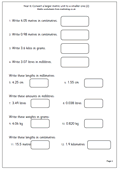This is about as hard as it gets for converting larger metric units to smaller ones. The larger units all include decimals and a good knowledge of multiplying by 10, 100 or 1000 is needed.

As a special treat the last question is really tricky; How many centimetres in 1.9 kilometres. I imagine that there are quite a lot of adults who would struggle with that one, but taken in logical steps it proves to be not so difficult. Find the number of metres in 1.9 kilometres, then find the number of centimtres in that amount. easy eh. It is quite interesting to look at other distances in terms of centimetres: journey home from school, length of a football pitch etc which would make for an interesting investigation.

Year 6 Convert larger metric units to smaller (pg 2)

## Maths puzzle: Initial numbers answers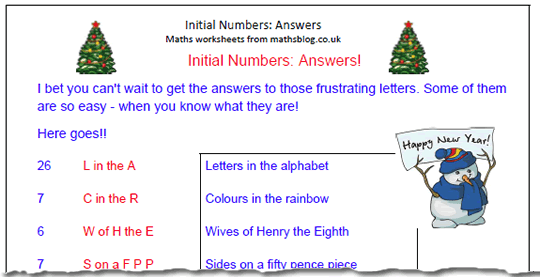I bet you can’t wait to get the answers to those frustrating letters I put on the site last week. Well, here they are. Some are very simple, such as:

28 days in February or 1066 Battle of Hastings.

## 01.10.01.10.01.10

Well it looks as if the Binary Age is almost upon us and we’ve been waiting a long time for this tremendous event.

Of course 1st January 2010 can be represented as 01.01.10, but New Year’s Day is just the start of something much bigger.

The 1st second of the 1st minute of the 1st hour of the 1st day of January 2010 can be written:

01.01.01.01.01.10

but perhaps even more interesting is a date later in the month: Continue reading “01.10.01.10.01.10”

## Maths puzzle: Initial Numbers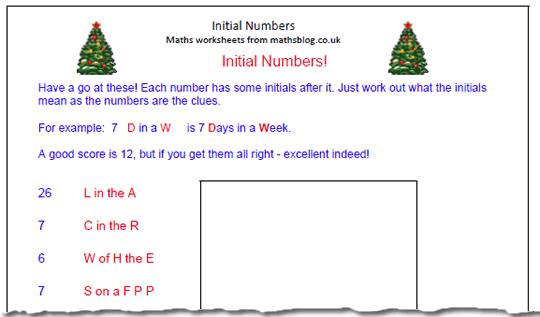It’s the last day of term and snowing quite heavily here so it looks as if there is no school at all for many children. Teachers will not be getting their pressies or having their last afternoon parties!

Oh well, here is a little puzzle for you to contemplate over the Christmas period.

Each number has some initials next to it. Just work out what the initials mean as the numbers are the clue.

For example:

7 D in a W is 7 Days in a Week

Some are these are pretty tricky and a score of 12 is good. Get them all right and you are a superstar indeed.

Maths Puzzle: initial numbers

## Maths Worksheet: Converting metric units (1)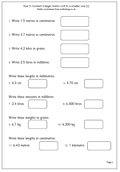The Metric system is so much easier than the old Imperial system, but there are still a few problems which come up when converting larger units to smaller units. Before attempting this certain facts need to be learnt off by heart, including;

10 mm = 1 cm

100 cm = 1 m

## Resource of the Week: Adding puzzle for Christmas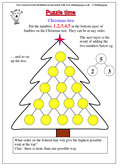Once again from the stats I can see that everyone is very busy with shopping, eating, drinking etc so will join you all and have a week off. I will leave you with a little puzzle given to us by Mathsphere which we sent up a year ago in case you have nothing better to do!

Put the numbers 1, 2, 3, 4, 5 in the bottom layer of baubles on the Christmas tree. The next layer is made by adding the pairs of numbers below and so on up to the top.

The numbers on the bottom row can be put in any order – what order will give the highest possible total at the top?

Answer is given for those who have eaten too many mince pies.

Happy Christmas

## Tables worksheet: 2, 5 and 10 times tables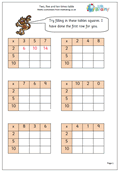Practice makes perfect, and this multiplication page gives loads of practice for the 2x, 5x and 10x tables. This is the first stage in introducing the idea of filling in a multiplication grid, multiplying the numbers across and down to fill in the grid.

The first time children come across this they will probably need some assistance, but once a couple have been completed they should be OK.

It is interesting to watch the techniques used to complete these grids. Some children proceed in strict order, others go for the easy ones first. It can be made more of a challenge to set a time limit for the whole page.

2x, 5x, 10x tables grids

## Time: Days, hours, minutes and seconds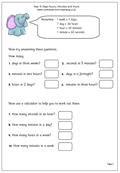By year 4 children are expected to know that:

1 week = 7 days
1 day = 24 hours
1 hour = 60 minutes
1 minute = 60 seconds

This maths worksheet uses this knowledge to answer  some straightforward questions. It also requires a calculator so more detailed information can be found, such as the number of minutes in a week. This, in turn, can lead on to some interesting investigative work, such as finding out how long a year is in minutes, and then how many minutes you have been alive (not forgetting leap years). It is quite an eye opener to realise how many precious minutes have been taken up in queues, traffic jams etc!!

This worksheet can be found in our Year 4 resources, under Measures

Days, hours, minutes and seconds

## Coming Soon: Time, the Metric System and tables.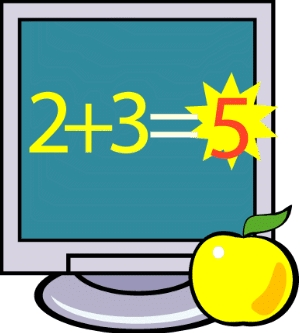By year 4 children are expected to know that:

1 week = 7 days
1 day = 24 hours
1 hour = 60 minutes
1 minute = 60 seconds

One of next week’s maths worksheets uses this knowledge to answer  some straightforward questions. It also requires a calculator so more detailed information can be found, such as the number of minutes in a week.

As we all know, practice makes perfect, and we are publishing a page next week which gives loads of practice for the 2x, 5x and 10x tables. This is the first stage in introducing the idea of filling in a multiplication grid, multiplying the numbers across and down to fill in the grid.

The Metric system is so much easier than the old Imperial system, but there are still a few problems which come up when converting larger units to smaller units.Look out for this great worksheet on converting metric units.

## Maths worksheet: Negative numbers on a number line (2)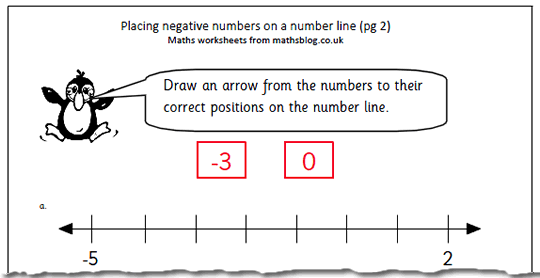This is the second page on  placing negative numbers on a number line, probably most suited to year 4 children. It asks for an arrow to be drawn to the correct position on the number line for each of the numbers shown.

Once again try to use the term ‘negative number’ rather than ‘minus’ which should be used in the context of subtraction.

Counting up and down a number line, passing through zero is a great way to show how negative numbers work and children will need to have done this before trying this worksheet.

Place negative numbers on a number line (2)